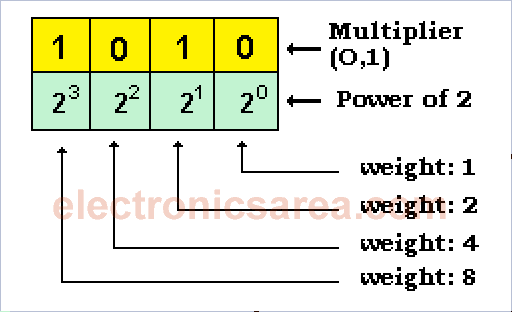# The Binary Number System (base 2)

The Binary number system, unlike the Decimal number System, where they are allowed 10 digits (0 to 9), you only need two (2) digits: “0” and “1”. The Binary number system is especially important in digital electronics, where only two values are possible: “1” or “high” and “0” or “low”.

“1” and “0” are associated with:

– “high level” and “low level”
– “closed” and “open”
– “on” and “off”
– “connected” and “disconnected”
– “high” and “low”, etc..

Analyzing the diagram below. A number on the binary numbering system, is divided into different weight digits: 1, 2, 4, 8, 16, 32, 64, 128, …. etc.Each weight is associated with a power of 2. On the first digit (from right to left) the power of 2 is 20, the second digit the power of 2 is 21 and we continue in the same way until the last number on the left. Then, to form the number 10102. Number 10 (decimal) in binary.•
•
•
•
•
•
•
•
•
•
•
•
•
•
•
•
•
•